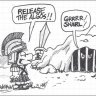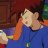# Useful Intraday Stats for ThinkorSwim

S

#### Sonny

##### Active member
VIP
Hi,

I would like to submit the following chart study code for intraday stock stats. I feel that this will be very helpful to investors as they can see the vital stock stats on the chart. Please pass this on! The code is attached below this.

Thanks,
SonnyCode:
``````#Current Price
Addlabel (1, "Price: " + close, color.CYAN);

#Percent Change from yesterday close
def AP = AggregationPeriod.DAY;
def Priorclose = close(period = AP);
def PctChange = (close - Priorclose) / Priorclose;
def PC1 = Round(PctChange,3);
Addlabel(1, "Ch: " + AsPercent(PC1), if PctChange > 0 then Createcolor(238,210,238) else Color.LIGHT_RED);

#Prior Close
Addlabel (1, "YC: " + Round(Priorclose,2), color.LIGHT_GREEN);

#Open price
def open1 = open(period = AP);
Addlabel (1, "O: " + Round(open1,2), createcolor(254,216,177));

#High of Day
def high1 = Highest(high(period = AP),1);
Addlabel (1, "Hi: " + Round(high1,2), color.CYAN);

#Low of Day
def low1 = Lowest(low(period = AP),1);
Addlabel (1, "Lo: " + Round(low1,2), createcolor(178,255,102));

#Volume Today
def today = volume(period = "DAY");
def L1 = RoundDown(lg(today),0);
Addlabel (L1 < 5, "Vol: " + today, createcolor(206,177,128));
Addlabel (L1 between 4.5 and 5.5, "Vol: " + Round((today/1000),0) + "K", createcolor(206,177,128));
Addlabel (L1 between 5.5 and 10, "Vol: " + Round((today/1000000),1) + "M", createcolor(206,177,128));

#Change From Open
def CFO = (close / open1)-1;
def CFO1 = Round(CFO,3);
Addlabel(1, "CFO: " + AsPercent(CFO1), if CFO >0 then Createcolor(0,255,128) else createcolor(255,153,153));``````

Last edited by a moderator:
C

#### convertiblejay

##### New member
is there a way you can add Yesterdays volume or thats to much to ask for? I like the indicator btw

S

#### Sonny

##### Active member
VIP
is there a way you can add Yesterdays volume or thats to much to ask for? I like the indicator btw

Just add the following code at the bottom for yesterday's volume:

#Yest Vol
def YV = volume(period = AP);
def YVL = RoundDown(lg(YV),0);
Addlabel (YVL < 5, "YV: " + YV, createcolor(173,216,230));
Addlabel (YVL between 4.5 and 5.5, "YV: " + Round((YV/1000),0) + "K", createcolor(173,216,230));
Addlabel (YVL between 5.5 and 10, "YV: " + Round((YV/1000000),1) + "M", createcolor(173,216,230));

•markos and mansor
R

S

#### Sonny

##### Active member
VIP

For Premarket scan, I look for Price between 0.3 and 10, Volume > 5000, and Study Filter {After hours percent change: Close greater than 10%}, which is listed under Price Performance.
For Market Hours scan, I look for Price between 0.3 and 10, Volume > 100000, and % change greater than 15%.

C

#### convertiblejay

##### New member
@Sonny Thank you brother you are appreciated

O

#### otmane

##### New member
hi sonny thanks for this code but what about it if i wanna see the whole volume number not just the first 4 numbers

S

#### Sonny

##### Active member
VIP
hi sonny thanks for this code but what about it if i wanna see the whole volume number not just the first 4 numbers

If you want the entire volume number for today's volume, just use the following code instead of the original volume code:

#Volume today
def today = volume(period = AP);
Addlabel(1, "Vol: " + today, createcolor(206,177,128));

•soary and convertiblejay
J

#### Johnny Cash

##### Member
Thanks Sonny, good work.
Is there a way to create a label that also shows futures on this chart?

S

#### Sonny

##### Active member
VIP
Thanks Sonny, good work.
Is there a way to create a label that also shows futures on this chart?
I don't think it's possible to add futures as a chart label.

•Johnny Cash
S

#### Sonny

##### Active member
VIP
Just add this code to get Premarket High as a chart label as well:

def h = high;
def bar = BarNumber();
def GlobeX = GetTime() < RegularTradingStart(GetYYYYMMDD());

def ONhigh = if GlobeX and !GlobeX then h else if GlobeX and h > ONhigh then h else ONhigh;
def ONhighBar = if GlobeX and h == ONhigh then bar else Double.NaN;
def OverNightHigh = if BarNumber() == HighestAll(ONhighBar) then ONhigh else OverNightHigh;
AddLabel(1, "PMH: " + Round(OverNightHigh,2), color.CYAN);

•markos and tenacity11
C

#### convertiblejay

##### New member
Hey @Sonny i have a question. Is there a way to show like what the difference between yesterdays volume vs todays (current day) ? like to say if it will pass the previous day volume or trend higher and pass it in current time instead of waiting till days end?

P

#### Pensar

##### Active member
VIP
Thanks Sonny, good work.
Is there a way to create a label that also shows futures on this chart?

@Johnny Cash - I took the original labels @Sonny wrote and edited them to allow a user-selected symbol. I did test it against the original version using large cap stocks, penny stocks, and futures, and it appeared to run correctly. Make sure it displays the correct info before you use it.

Code:
``````#Intraday Stats
#Sonny @ usethinkscript.com

input symbol = "/ES";
def c = close(symbol, aggregationperiod.DAY);
def o = open(symbol, aggregationperiod.DAY);
def h = high(symbol, aggregationperiod.DAY);
def l = low(symbol, aggregationperiod.DAY);
def v = volume(symbol, aggregationperiod.DAY);

#Symbol Label

#Current Price
Addlabel (1, "Price: " + c, color.CYAN);

#Percent Change from yesterday close
def Priorclose = c;
def PctChange = (c - Priorclose) / Priorclose;
def PC1 = Round(PctChange,3);
Addlabel(1, "Ch: " + AsPercent(PC1), if PctChange > 0 then Createcolor(238,210,238) else Color.LIGHT_RED);

#Prior Close
Addlabel (1, "YC: " + Round(Priorclose,2), color.LIGHT_GREEN);

#Open price
Addlabel (1, "O: " + Round(o,2), createcolor(254,216,177));

#High of Day
def high1 = Highest(h,1);
Addlabel (1, "Hi: " + Round(high1,2), color.CYAN);

#Low of Day
def low1 = Lowest(l,1);
Addlabel (1, "Lo: " + Round(low1,2), createcolor(178,255,102));

#Volume Today
def L1 = RoundDown(lg(v),0);
Addlabel (L1 < 5, "Vol: " + v, createcolor(206,177,128));
Addlabel (L1 between 4.5 and 5.5, "Vol: " + Round((v/1000),0) + "K", createcolor(206,177,128));
Addlabel (L1 between 5.5 and 10, "Vol: " + Round((v/1000000),1) + "M", createcolor(206,177,128));

#Change From Open
def CFO = (c / o)-1;
def CFO1 = Round(CFO,3);
Addlabel(1, "CFO: " + AsPercent(CFO1), if CFO >0 then Createcolor(0,255,128) else createcolor(255,153,153));

#end code``````

Last edited:
•markos
S

#### Sonny

##### Active member
VIP
Hey @Sonny i have a question. Is there a way to show like what the difference between yesterdays volume vs todays (current day) ? like to say if it will pass the previous day volume or trend higher and pass it in current time instead of waiting till days end?

If want to know the difference between today's volume and yesterday's volume, you could add the following code:

def difvol = today-YV;

Make sure you add the code for yesterday's volume which I gave above before adding this code for difvol.

•soary
C

#### convertiblejay

##### New member
If want to know the difference between today's volume and yesterday's volume, you could add the following code:

def difvol = today-YV;

Make sure you add the code for yesterday's volume which I gave above before adding this code for difvol.

Thank you so much#### markos

##### Well-known member
VIP
@Sonny & @Pensar Thank you kindly for the Label Codes!
Before the question is asked, would either of you want to show everyone how use these in a watch list?

S

#### Sonny

##### Active member
VIP
@Sonny & @Pensar Thank you kindly for the Label Codes!
Before the question is asked, would either of you want to show everyone how use these in a watch list?

markos:

These chart labels can only be used as a chart study to track stats on a specific stock. If you want these same stats for a watchlist, you can just click on Customize at the far right top corner of the list and add these columns. The previous close will be Yesterday Close and the only column you need to create will be Change From Open % (CFO). For CFO, add this code to a Custom formula and label it:

#Percent change from open
plot x = Round(100*((close/open)-1),1);
x.assignValueColor( if x < 0 then color.RED else color.GREEN);

Hope that clears things up and let me know if you have more questions.

Sonny

•markos
S

#### Sonny

##### Active member
VIP
I modified my original code so that the intraday labels will show up only at 9:30am. From 4am to 9:30am, you will get Current price, Percent Change, Yesterday Close, and Premarket High (PMH). The PMH will disappear at 10am. The key to the labels appearing is the first variable in Addlabel. The label will show if it is 1 and not show if it is 0.

Code:
``````#LABELS
#
#Current Price
AddLabel (1, "P: " + Round(close,2), Color.CYAN);

#Percent Change from yesterday close
def AP = AggregationPeriod.DAY;
def Priorclose = close(period = AP);
def PctChange = (close - Priorclose) / Priorclose;
def PC1 = Round(PctChange, 3);
AddLabel(1, "Ch: " + AsPercent(PC1), if PctChange > 0 then CreateColor(238, 210, 238) else createcolor(255,153,153));

#Check if time is premarket
def pmopen = if secondsFromTime(0400)>=0 and secondstillTime(0930)>=0 then 1 else 0;
def pmclosed = if secondsFromTime(0400)>=0 and secondstillTime(0930)>=0 then 0 else 1;
def pastten = if secondsFromTime(0400)>=0 and secondstillTime(1000)>=0 then 1 else 0;

#Prior Close
AddLabel (1, "YC: " + Round(Priorclose, 2), Color.LIGHT_GREEN);

#Open price
def open1 = open(period = AP);
AddLabel (pmclosed, "O: " + Round(open1, 2), CreateColor(254, 216, 177));

#High of Day
def high1 = Highest(high(period = AP), 1);
AddLabel (pmclosed, "H: " + Round(high1, 2), Color.CYAN);

#Low of Day
def low1 = Lowest(low(period = AP), 1);
AddLabel (pmclosed, "L: " + Round(low1, 2), CreateColor(178, 255, 102));

#Volume Today
def today = volume(period = AP);
def L1 = RoundDown(Lg(today), 0);
AddLabel (L1 < 5, "V: " + today, CreateColor(206, 177, 128));
AddLabel (L1 between 4.5 and 5.5, "V: " + Round((today / 1000), 0) + "K", CreateColor(206, 177, 128));
AddLabel (L1 > 5, "V: " + Round((today / 1000000), 1) + "M", CreateColor(206, 177, 128));

#Change From Open
def CFO = (close / open1) - 1;
def CFO1 = Round(CFO, 3);
AddLabel(pmclosed, "C: " + AsPercent(CFO1), if CFO > 0 then CreateColor(0, 255, 128) else CreateColor(255, 153, 153));

def h = high;
def bar = BarNumber();
def GlobeX = GetTime() < RegularTradingStart(GetYYYYMMDD());

def ONhigh = if GlobeX and !GlobeX then h else if GlobeX and h > ONhigh then h else ONhigh;
def ONhighBar = if GlobeX and h == ONhigh then bar else Double.NaN;
def OverNightHigh = if BarNumber() == HighestAll(ONhighBar) then ONhigh else OverNightHigh;
AddLabel(pastten, "PMH: " + Round(OverNightHigh,2), color.CYAN);``````

•S

#### Sonny

##### Active member
VIP
@teo_teo asked for the following chart labels:

Premarket gap %
PM gap down (from PM High)
Open to current High as %
High to current price

If you look at the code I just posted above this, you will see PM gap % as the percent change.

The PM gap down (from PM high) would show up with this code:

def PMGD = close - OverNightHigh;
Addlabel(pmopen, "PMGD: " + PMGD, color.PINK);

Open to current High as % would show up with this code:

def OH1 = Round(100*((high1/open1)-1),1);
Addlabel(pmclosed, "OH1: " + OH1 + "%", createcolor(48,138,183));

High to current price would show up with this:

def HC = close - high1;
Addlabel(pmclosed, "HC: " + HC, createcolor(217,168,215));

Make sure these codes are added to the bottom of the big code I just posted above.

Last edited:
•soary, markos and teo_teo
H

#### henry1224

##### Member
VIP
@Pensar I keep getting an error message with change from Open

•PensarUseful Candlestick Studies Indicators 17